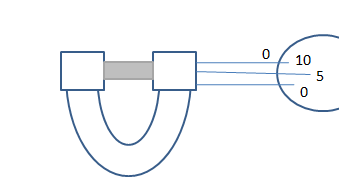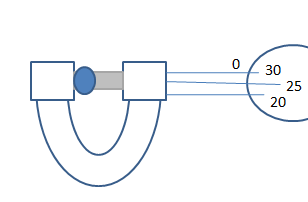Courses
Courses for Kids
Free study material
Offline Centres
MoreLast updated date: 28th Nov 2023
Total views: 279.3k
Views today: 3.79k

# The circular divisions of the shown screw gauge are$50$ . It moves $0.5mm$ on the main scale in one rotation. The diameter of the ball is:$(A)2.25mm \\ (B)2.20mm \\ (C)1.20mm \\ (D)1.25mm \\$Verified
279.3k+ views
Hint: Find the formula of zero error of a screw gauge and calculate it with the given data.
Also, calculate the least count of the circular scale used in the screw gauge.
Using the least count, find the main scale reading and circular scale reading.
Note that the formula of actual measurement is based upon these two readings and the zero error of the screw gauge.

From the 1st figure, the Zero error of the screw gauge is, $Z.E = \dfrac{{5 \times 0.5}}{{50}} = 0.05mm$
Now the least count of the circular scale is, $L.C = \dfrac{{0.5}}{{50}} = 0.01mm$
So, The circular scale reading, $C.S.R = 25 \times 0.01 = 0.25mm$
And, the main scale reading, $M.S.R = 2 \times 0.5 = 1mm$
So the diameter will be C. S.R+M.S.R-Z.E
Hence the diameter = $0.25 + 1 - 0.05 = 1.20mm$
So, the correct answer is “Option C”.